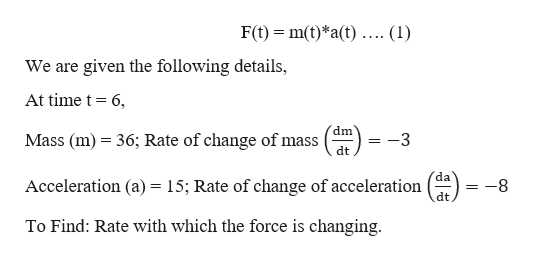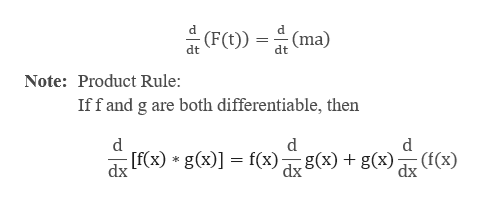# F(t)=m(t)a(t)At time t=6 seconds, the mass of an object is 36 grams and changing at a rate of −3(g/s). At this same time, the acceleration is 15(m/s^2) and changing at a rate of −8(m/s^3)By the product rule, the force on the object is changing at the rate of...?

Question
3 views

F(t)=m(t)a(t)

At time t=6 seconds, the mass of an object is 36 grams and changing at a rate of −3(g/s). At this same time, the acceleration is 15(m/s^2) and changing at a rate of −8(m/s^3)

By the product rule, the force on the object is changing at the rate of...?

check_circle

Step 1

The force on an object is the product of the mass m and the acceleration. In this problem, we assume both mass and acceleration are depended on time t.help_outlineImage TranscriptioncloseF(t)m(t)*a(t).... (1) = We are given the following details, At time t 6, dm Mass (m) 36; Rate of change of mass dt da Acceleration (a) = 15; Rate of change of acceleration dt -8 To Find: Rate with which the force is changing fullscreen
Step 2

To determine the rate of change, we differentiate the force with respect to time (t).help_outlineImage Transcriptionclosed (F(t)) (ma) dt dt Note: Product Rule: Iffand g are both differentiable, then d d [f(x) g(x)f(x) g(x) g(x) (f(x) dx dx fullscreen
Step 3

Substitute the val...

### Want to see the full answer?

See Solution

#### Want to see this answer and more?

Solutions are written by subject experts who are available 24/7. Questions are typically answered within 1 hour.*

See Solution
*Response times may vary by subject and question.
Tagged in

### Derivative